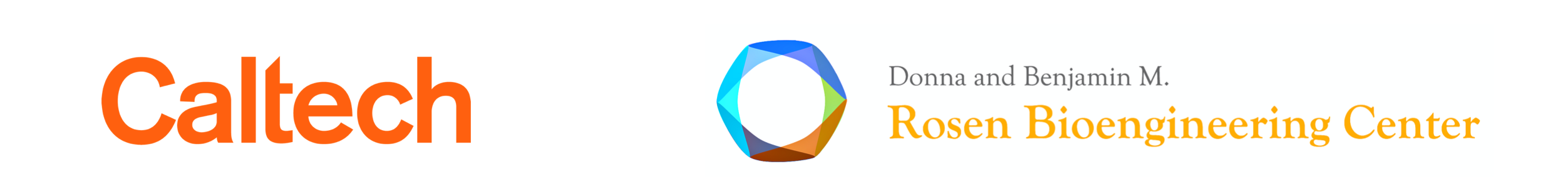# Lesson 38: Review of exercise 5¶

This document was prepared at Caltech with financial support from the Donna and Benjamin M. Rosen Bioengineering Center.This lesson was generated from a Jupyter notebook. You can download the notebook here.

In :
import os
import glob

import numpy as np
import pandas as pd

import skimage.io
import skimage.morphology
import skimage.segmentation
import skimage.measure

import bokeh_catplot
import bootcamp_utils

import bokeh.io
bokeh.io.output_notebook()


## Problem 5.0¶

Do any of the previous exercises you have not completed and are interested in working on.

## Problem 5.1: Growth curves from a movie¶

At the dawn of the molecular revolution in biology, key experiments by Jacques Monod in which he measured growth curves of bacteria under different conditions exposed some of the mechanisms of regulation of gene expression. Those growth curves were measured in a bulk solution. In this exercise, we will measure bacterial growth starting from two bacteria. The movie shows Bacillus subtilis constitutively expressing mCherry growing under slow growth conditions. These data were kindly donated by Jin Park from the Elowitz lab at Caltech.

a) Load in the series of images contained in the directory ~git/bootcamp/data/bacterial_growth/. Be sure that however you store them (a list or tuple or other object) has the frames in the proper order.

b) Segment the images to separate bacteria from background. You do not need to segment individual bacteria; this would likely require some more advanced techniques involving edge detection that we haven't covered in bootcamp.

c) Show a representative image from the stack (with the segmentation overlayed) of images. Be sure to check out the README file in the directory containing the images to get the interpixel distance.

d) If $m$ is the mass of bacteria, which is proportional to the area in the images, then, for exponential growth,

\begin{align} m(t) = m_0\,\mathrm{e}^{rt}, \end{align}

where $r$ is the growth rate. Taking the logarithm of both sides gives

\begin{align} \ln m(t) = \ln m_0 + rt. \end{align}

So, the slope of the line on a log-log scale is the growth rate.

With this in mind, plot the bacterial area as a function of time with the area on a log scale. If the plot is linear, the bacteria are performing exponential growth.

e) To get the slope of the line, you can use the np.polyfit() function and perform the regression on a semilog scale. Alternatively, you can fit the exponential function directly using scipy.optimize.curve_fit(). Once you find the growth rate (and intercept), plot the theoretical growth curve along with the data.

### Problem 5.1: solution¶

a) We will load the images into a tuple, where the index of the tuple corresponds to the frame number. Under normal circumstances, we would do data validation to make sure there are no skipped frames, but in the interest of brevity, we will proceed assuming all data are properly labeled and organized.

In :
# Use a list comprehension to read in the images
for fname in sorted(glob.glob('data/bacterial_growth/bacillus_*.tif'))]

# Store it as a tuple so we don't mess with it
ims = tuple(ims)

# How many?
print('There are', len(ims), 'images.')

There are 55 images.


Let's take a quick look at a few of the images. We'll look at frames 0, 15, 30, and 45. For now, we will not worry about setting the scales of the axes.

In :
plots = [bootcamp_utils.bokeh_imshow(ims[i], plot_height=300) for i in [0, 15, 30, 45]]

bokeh.io.show(bokeh.layouts.gridplot(plots, ncols=2))